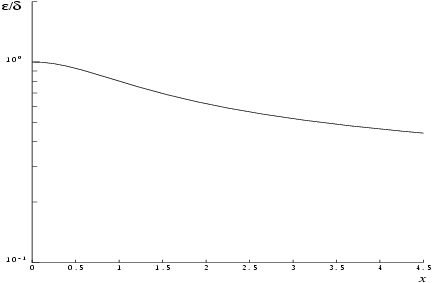NAG Library Function Document

1Purpose

nag_arcsinh (s11abc) returns the value of the inverse hyperbolic sine, $\mathrm{arcsinh}x$.

2Specification

 #include #include
 double nag_arcsinh (double x)

3Description

nag_arcsinh (s11abc) calculates an approximate value for the inverse hyperbolic sine of its argument, $\mathrm{arcsinh}x$.
For $\left|x\right|\le 1$ it is based on the Chebyshev expansion
 $arcsinh⁡x=x×yt=x∑′r=0crTrt, where ​t=2x2-1.$
For $\left|x\right|>1$ it uses the fact that
 $arcsinh⁡x=sign⁡x×lnx+x2+1 .$
This form is used directly for $1<\left|x\right|<{10}^{k}$, where $k=n/2+1$, and the machine uses approximately $n$ decimal place arithmetic.
For $\left|x\right|\ge {10}^{k}$, $\sqrt{{x}^{2}+1}$ is equal to $\left|x\right|$ to within the accuracy of the machine and hence we can guard against premature overflow and, without loss of accuracy, calculate
 $arcsinh⁡x=sign⁡x×ln⁡2+lnx.$
Abramowitz M and Stegun I A (1972) Handbook of Mathematical Functions (3rd Edition) Dover Publications

5Arguments

1:    $\mathbf{x}$doubleInput
On entry: the argument $x$ of the function.

None.

7Accuracy

If $\delta$ and $\epsilon$ are the relative errors in the argument and the result, respectively, then in principle
 $ε≃ x 1+x2 arcsinh⁡x δ .$
That is, the relative error in the argument, $x$, is amplified by a factor at least $\frac{x}{\sqrt{1+{x}^{2}}\mathrm{arcsinh}x}$, in the result.
The equality should hold if $\delta$ is greater than the machine precision ($\delta$ due to data errors etc.) but if $\delta$ is simply due to round-off in the machine representation it is possible that an extra figure may be lost in internal calculation round-off.
The behaviour of the amplification factor is shown in the following graph:Figure 1
It should be noted that this factor is always less than or equal to one. For large $x$ we have the absolute error in the result, $E$, in principle, given by
 $E∼δ.$
This means that eventually accuracy is limited by machine precision.

8Parallelism and Performance

nag_arcsinh (s11abc) is not threaded in any implementation.

None.

10Example

This example reads values of the argument $x$ from a file, evaluates the function at each value of $x$ and prints the results.

10.1Program Text

Program Text (s11abce.c)

10.2Program Data

Program Data (s11abce.d)

10.3Program Results

Program Results (s11abce.r)

© The Numerical Algorithms Group Ltd, Oxford, UK. 2017